• ## 机器人运动学逆解

千次阅读 2019-11-24 10:44:10
机器人运动学逆解 即根据工具坐标系相对于基坐标系的目标位姿，求解机器人各关节角。逆运动学在机器人学中占有非常重要的地位，是机器人轨迹规划和运动控制的基础，直接影响着控制的快速性与准确性。一般机器人运动...
机器人运动学逆解
即根据工具坐标系相对于基坐标系的目标位姿，求解机器人各关节角。逆运动学在机器人学中占有非常重要的地位，是机器人轨迹规划和运动控制的基础，直接影响着控制的快速性与准确性。一般机器人运动学逆解算法可分为以下几种: 解析法 ( 又称反变换法) 、几 何 法 和 数 值 解法。由 PAUL 等提出的反变换法求解过程直观，因而被广泛采纳，但其求解过程中需多次进行齐次变换矩阵的逆运算和 4 × 4 维矩阵的乘积运算，导致求解过程复杂耗时。


展开全文• 一般机器人运动学逆解算法可分为以下几种: 解析法 ( 又称反变换法) 、几 何 法 和 数 值 解法。由 PAUL 等提出的反变换法求解过程直观，因而被广泛采纳，但其求解过程中需多次进行齐次变换矩阵的逆运算和 4 × 4 维...
为什么要运动学逆解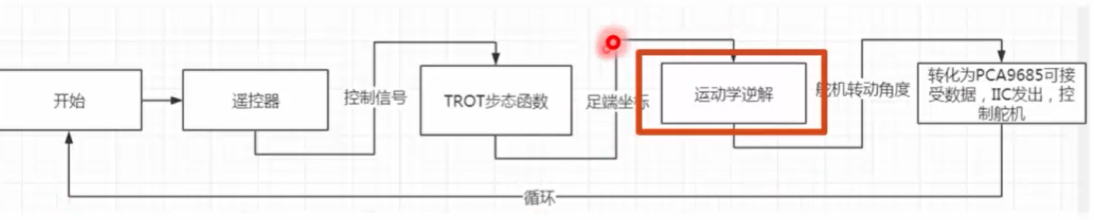运动学逆解即根据工具坐标系相对于基坐标系的目标位姿，求解机器人各关节角。
逆运动学在机器人学中占有非常重要的地位，是机器人轨迹规划和运动控制的基础，直接影响着控制的快速性与准确性。一般机器人运动学逆解算法可分为以下几种: 解析法 ( 又称反变换法) 、几 何 法 和 数 值 解法。由 PAUL 等提出的反变换法求解过程直观，因而被广泛采纳，但其求解过程中需多次进行齐次变换矩阵的逆运算和 4 × 4 维矩阵的乘积运算，导致求解过程复杂耗时。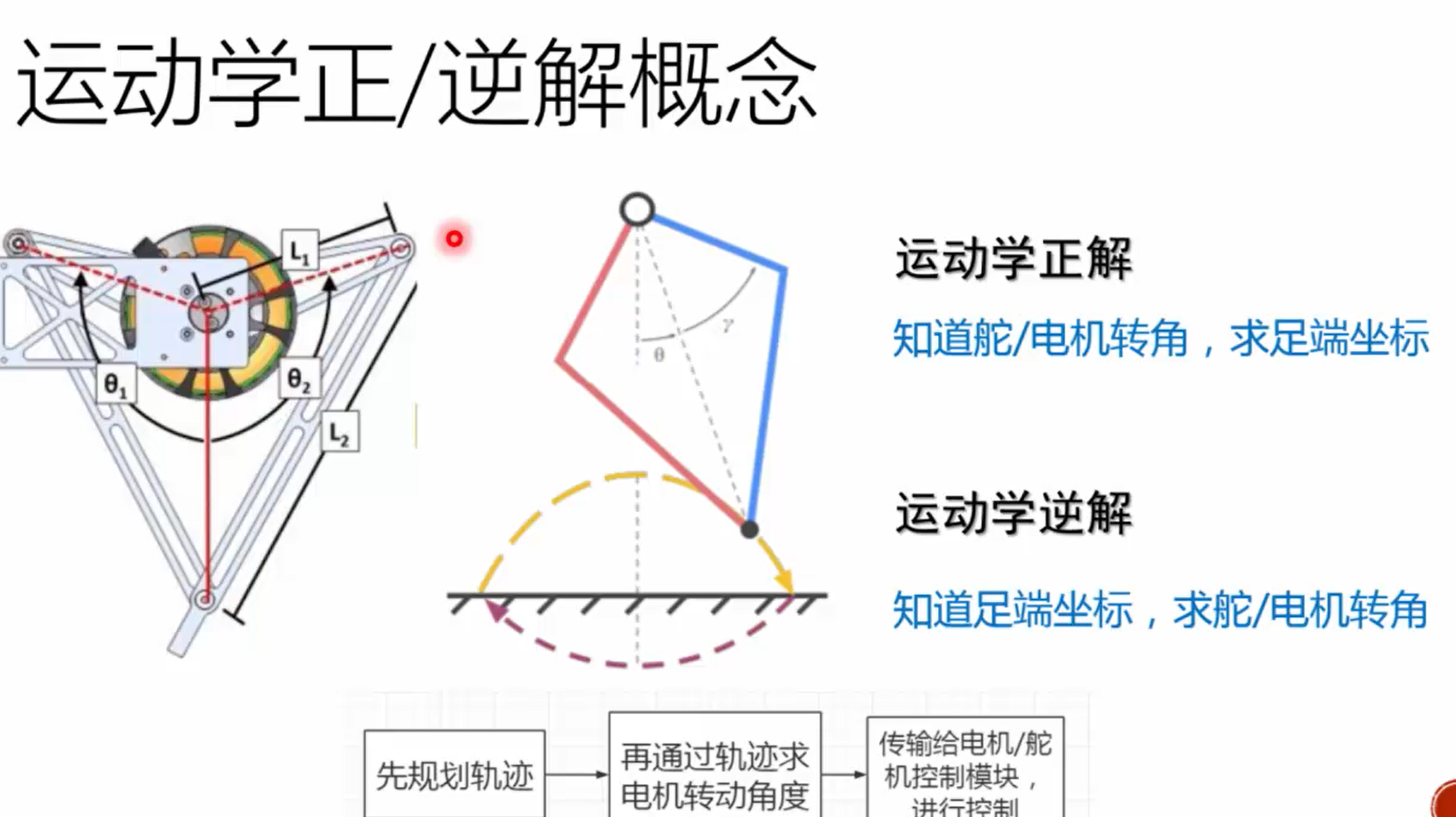足端轨迹规划
在机器人技术各个环节中，信息存在多样性，机器人作业也存在多样性。唯有机器人轨迹规划存在唯一性，机器人的使用都离不开轨迹规划与运动轨迹。随着现在机器人技术的发展，运动规划的原理已慢慢形成共识，对于关节规划逐渐存在主流技术和解决方案。主要会在避障等额外要求下做出规划需求。所以看似不起眼的机器人轨迹规划反而是所有机器人作业中绕不开的一个过程。而且轨迹规划是没有主流要求而言的。按照不同的要求需要不同的轨迹。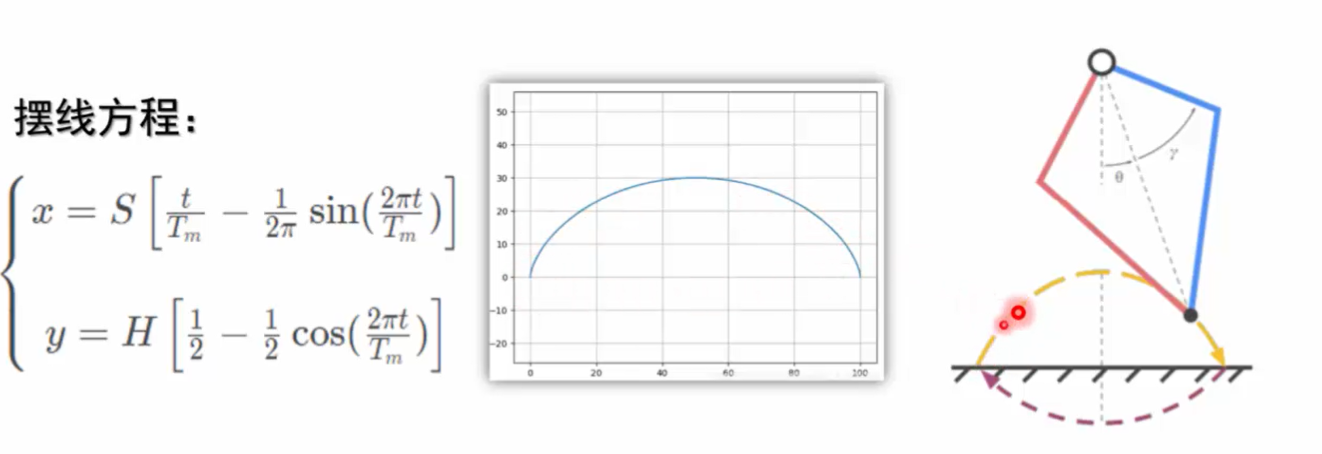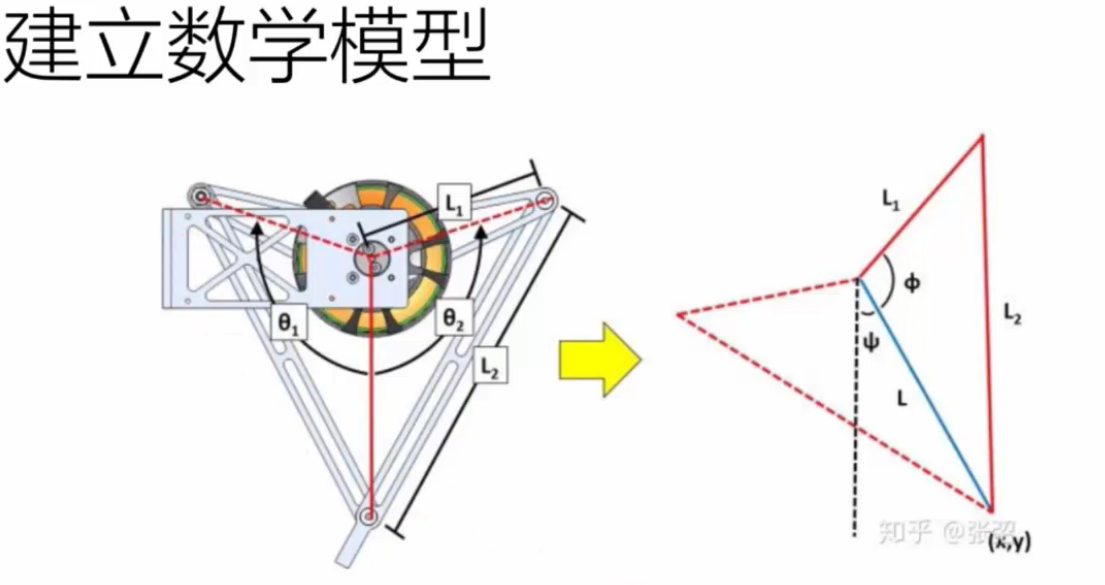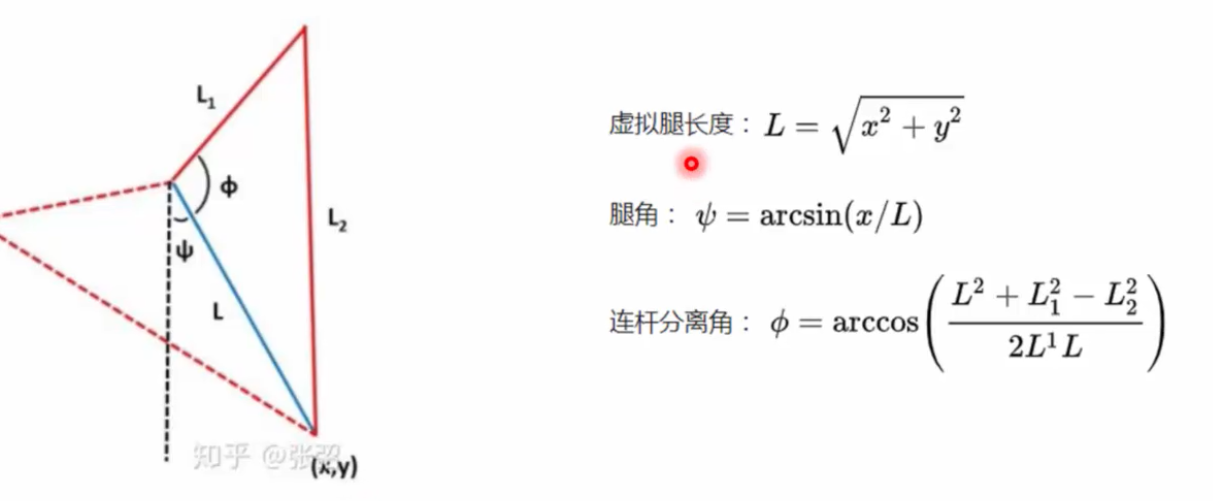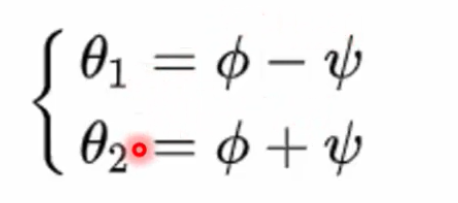课后题求逆解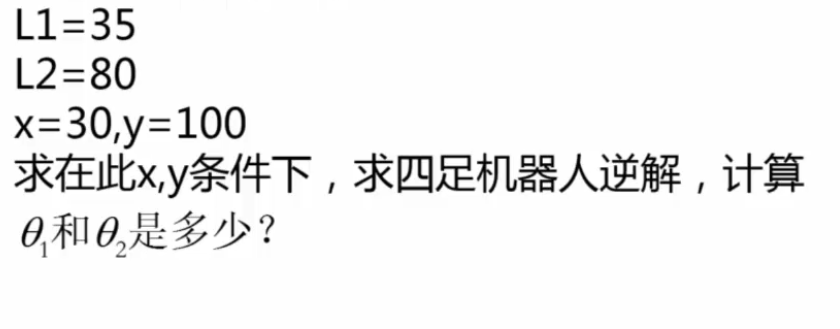解题方法
import math

def fun(x,y,l1,l2):
l=math.sqrt(x*x+y*y)#求虚拟脚长度
psail=math.asin(x/l)#脚角
fail=math.acos((l*l+l1*l1-l2*l2)/(2*l*l1))#连杆分离角
m1=fail-psail#右舵机弧度制
m2=fail+psail#左舵机弧度制
sita1=180*m1/math.pi#右舵机角度制
sita2=180*m2/math.pi#左舵机角度制
return(sita1,sita2)

print(fun(30,100,35,80))

将 L1=35,L2=80,x=30,y=100 输入算法
求得：
右舵机弧度制 0.37927814199991783
左舵机弧度制 0.962191730955652
右舵机角度制 21.731036798158822
左舵机角度制 55.12952526614607
南昌理工学院人工智能学院特种机器人研发中心实验室workshop项目实践平台


展开全文•   今天，讲讲机器人运动学逆解中的一大“法宝”，最常用的三角方程： k1sin(θ)+k2cos(θ)=k3(1) k_1sin(\theta)+k_2cos(\theta)=k_3 \tag{1} k1​sin(θ)+k2​cos(θ)=k3​(1)   联立三角恒等式： sin2(θ)+cos...
文章目录一、推导步骤1、方法12、方法2二、实例(以SCARA机器人逆解为例)
在推导机器人运动学逆解的解析解时，经常会遇到以下三角方程：
$k_1sin(\theta)+k_2cos(\theta)=k_3 \tag{1}$
求解以上三角方程的解析解对运动学逆解的推导过程至关重要。下面采用两种方法进行推导。
一、推导步骤
1、方法1
式(1)联立以下三角恒等式：
$sin^2(\theta)+cos^2(\theta)=1 \tag{2}$
利用MATLAB解符号方程组(1)(2)，代码如下：
clc;
clear;
syms k1 k2 k3 theta sinTheta cosTheta real
result = solve(k1 * sinTheta + k2 * cosTheta == k3, sinTheta^2 + cosTheta^2 == 1, [sinTheta, cosTheta]);
sinTheta = simplify(result.sinTheta)
cosTheta = simplify(result.cosTheta)

很容易求得两组解：
$\begin{cases} sin\theta= (k_1k_3 \mp k_2\sqrt{k_1^2 + k_2^2 - k_3^2})/(k_1^2 + k_2^2) \\ cos\theta=(k_2k_3 \pm k_1\sqrt{k_1^2 + k_2^2 - k_3^2})/(k_1^2 + k_2^2) \\ \tag 3 \end{cases}$
若$k_1^2+k_2^2，则当前给定位姿区域不可到达；若$k_1=k_2=0$，则当前给定位姿为奇异位姿。这里，采用代数的方法便可方便判断给定位姿是否在机器人可达工作空间里，并判断该位姿是否为奇异位姿！
若$k_1^2+k_2^2\ge k_3^2$，由于$k_1^2+k_2^2>0$，易得：
$\theta=atan2(k_1k_3 \mp k_2\sqrt{k_1^2 + k_2^2 - k_3^2},k_2k_3 \pm k_1\sqrt{k_1^2 + k_2^2 - k_3^2}) \tag{4}$
这里采用双变量反正切函数$atan2$，有几个优点，详见博文:为什么机器人运动学逆解最好采用双变量反正切函数atan2而不用反正/余弦函数？
2、方法2
令$tan(\theta/2)=u$，将三角恒等式$cos\theta=(1-u^2)/(1+u^2),sin\theta=2u/(1+u^2)$代入式(1)，整理得：
$(k_2+k_3)u^2-2k_1u+k_3-k_2=0 \tag{5}$
当$k_2+k_3=0$时，$\theta=\pm \pi$。
当$k_2+k_3\ne0$时，若$k_1^2+k_2^2，则当前给定位姿区域不可到达；
若$k_1^2+k_2^2\ge k_3^2$，求解式(5)关于$u$的一元二次方程的两实根，得到三角方程(1)的解：
$\theta= 2atan((k_1 \pm \sqrt{k_1^2+k_2^2-k_3^2})/(k_2+k_3))\tag{6}$
二、实例(以SCARA机器人逆解为例)
在博文：scara机器人运动学正逆解中，采用几何法推导得到机器人的运动学逆解，本文采用代数法来求运动学逆解。
SCARA机器人的运动学正解为：
$\left \{ \begin{array}{c} x=L_1cos\theta_1+L_2cos(\theta_1+\theta_2) \\ \tag 7 y=L_1sin\theta_1+L_2sin(\theta_1+\theta_2) \end{array}\right.$
式(5)可消去$sin(\theta_1+\theta_2),cos(\theta_1+\theta_2)$，转化为关于$\theta_1$的三角方程：
$2yL_1sin\theta_1+2xL_1cos\theta_1=x^2+y^2+ L_1^2-L_2^2\tag{8}$
令$k_1=2yL_1,k_2=2xL_1,k_3=x^2+y^2+ L_1^2-L_2^2$,可解得：
$\theta_1=atan2(k_1k_3 \mp k_2\sqrt{k_1^2 + k_2^2 - k_3^2},k_2k_3 \pm k_1\sqrt{k_1^2 + k_2^2 - k_3^2}) \tag{9}$
由式(5)得：
$\left \{ \begin{array}{c} sin(\theta_1+\theta_2)=(y-L_1sin\theta_1)/L_2 \\ \tag {10} cos(\theta_1+\theta_2)=(x-L_1cos\theta_1)/L_2 \end{array}\right.$
解得：
$\theta_2=atan2(y-L_1sin\theta_1,x-L_1cos\theta_1)-\theta_1\tag{11}$
MATLAB代码：
clc;
clear;
L1 = 200.0; %mm
L2 = 300.0; %mm
x = L1 * cos(theta1) + L2 * cos(theta1 + theta2);
y = L1 * sin(theta1) + L2 * sin(theta1 + theta2);
disp(['输入x，y(mm):',num2str(x), ',', num2str(y)])

k1 = 2.0 * y * L1;
k2 = 2.0 * x * L1;
k3 = x^2 + y^2 + L1^2 - L2^2;
temp = k1^2 + k2^2 - k3^2;
if temp < -eps
disp('区域不可到达');
return;
elseif temp < eps
disp('奇异点');
else
%do nothing
end
theta1 = zeros(2, 1);
theta1(1) = atan2(k1 * k3 - k2 * sqrt(temp), k2 * k3 + k1 * sqrt(temp));
theta1(2) = atan2(k1 * k3 + k2 * sqrt(temp), k2 * k3 - k1 * sqrt(temp));

s1 = sin(theta1);
c1 = cos(theta1);
theta2 = atan2(y - L1 * s1, x - L1 * c1) - theta1;
for i = 1 : 2
if theta2(i) > pi
theta2(i) = theta2(i) - 2.0 * pi;
end
if theta2(i) < -pi
theta2(i) = theta2(i) + 2.0 * pi;
end
end
xx = L1 * cos(theta1) + L2 * cos(theta1 + theta2);
yy = L1 * sin(theta1) + L2 * sin(theta1 + theta2);
disp(['输出角度(rad):',num2str(theta1(1)), ',', num2str(theta2(1)), '; ', num2str(theta1(2)), ',', num2str(theta2(2))])
disp(['输出x，y(mm):',num2str(xx(1)), ',', num2str(yy(1)), '; ', num2str(xx(2)), ',', num2str(yy(2))])



展开全文• 文章目录一、采用atan2(y,x)atan2(y,x)atan2(y,...  机器人运动学逆解最好采用双变量反正切函数atan2atan2atan2而不用反正/余弦函数asinasinasin和acosacosacos的原因主要有：   111.反正弦函数asin(x)asin(x)as...
文章目录一、采用$atan2(y,x)$的三点优势二、参考文献/资料
一、采用$atan2(y,x)$的三点优势
机器人运动学逆解最好采用双变量反正切函数$atan2$而不用反正/余弦函数$asin$和$acos$的原因主要有：
$1$.反正弦函数$asin(x)$的值域为$[-\pi/2,\pi/2]$，反余弦函数$acos(x)$的值域为$[0,\pi]$，而双变量反正切函数$atan2(y,x)$的值域为$[-\pi,\pi]$。机器人关节角度范围一般在$[-\pi,\pi]$之间，采用$atan2(y,x)$更加方便、直接，避免了额外的角度范围判断。
$2$.$atan2(y,x)$相对于$asin(x)$或$acos(x)$，对输入变量$x$、$y$具有更好的容错性。这里的容错性，主要是指由于计算精度的影响，$x$的实际计算值有可能稍微大于1或小于-1，这时$asin(x)$或$acos(x)$的值是未定义的，而$atan2(y,x)$可以得到正确的结果。当然，我们也可以通过判断$x$的值在计算误差范围内是否可以认为是-1或1，强制赋值为-1或1，从而解决这个问题。
$3$.对于函数$y=f(x)$，$x$的误差$\Delta x$引起$y$的误差为$\Delta y\approx f'(x)\Delta x$。
若$f(x)=asin(x)$，当$x\in(-1,1)$时，$f'(x)=\frac{1}{\sqrt{1-x^2}}\in[1,+\infty)$
若$f(x)=acos(x)$，当$x\in(-1,1)$时，$f'(x)=\frac{-1}{\sqrt{1-x^2}}\in(-\infty,-1]$
若$f(x)=atan(x)$，当$x\in(-\infty,+\infty)$时，$f'(x)=\frac{1}{x^2+1}\in(0,1]$
由于实际机器人的臂长、零点、减速比等运动学参数有误差，使用$asin(x)$或$acos(x)$误差会放大，从保证逆解精度均匀性来看，在求解机器人运动学逆解时宜采用$atan2(y,x)$。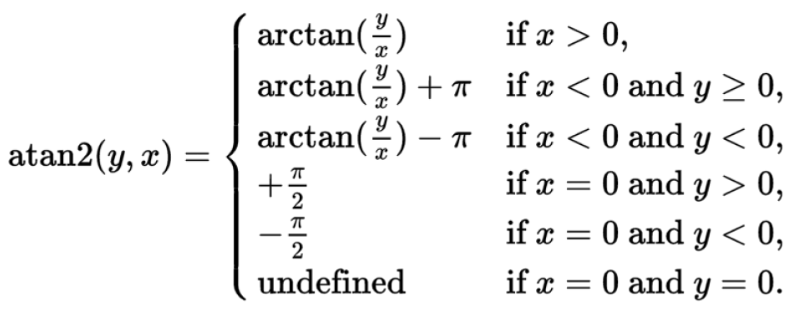二、参考文献/资料
机器人动力学与控制 霍伟


展开全文• 2.运动学逆解:已知足端坐标，求舵机/电机转角。 二.足端轨迹规划 摆线方程: { x=r*(t-sint) y=r*(1-cost) [其中r为圆的半径，t是圆的半径所经过的弧度（滚动角），当t由0变到2π时，动点就画出了摆线的一支，称为一...
• 以后将会给大家讲解如何手写正逆解以及轨迹插补的程序。程序是基于Matlab2016a，工具箱版本为Robotic Toolbox 9.10。1.D-H建模三个两两相互垂直的XYZ轴构成欧几里得空间，存在六个自由度：沿XYZ平移的三个自由度，绕...
• ## scara机器人运动学正逆解

千次阅读 多人点赞 2020-03-14 14:59:02
文章目录一、scara机器人运动学正解二、scara机器人运动学逆解1、正装scara机器人运动学逆解2、吊装scara机器人运动学逆解三、MATLAB代码 一、scara机器人运动学正解   末端BBB的xxx坐标为向量OA\bf{OA}OA与向量...
• 基于改进DH参数的机器人正解和逆解程序，逆解采用解析解形式，输出8组关节角度解
• Delta Robot Kinematics 并联机器人 运动学 正解 逆解 本人自己总结 matlab亲测 正反解正确
•研究论文
• ## 6轴机器人运动学正解,逆解1

万次阅读 多人点赞 2017-12-14 21:27:35
正解与逆解的求解需要相应的机器人运动方程，其中关键的就是DH参数表 DH参数表用来描述机器人各关节坐标系之间的关系，有了DH参数表就可以在机器人各关节之间进行坐标转换 求解正解就是从关节1到关节5的坐标转换 ...DH参数
• 六自由度机器人机械手运动学逆问题反程序。。。。
• 逆解 逆解计算方法可以参考以下书籍 机器人学导论——分析、系统及应用 电子工业出版社 机器人学导论第3版 机械工业出版社 .../*计算逆解 根据机器人坐标计算机器人关节角度 *关节参数在文件 param_table中 *...
• 导出了冗余度机器人基于优化力度的运动学逆解，并将优化力度函数用于冗余度机器人运动学优化控制。通过调整优化力度函数中自运动限制因子的取值，可以改变自运动速度在关节角速度中所占份额，实现人为地控制任务时间...
• 运动学逆解是指已知机器人末端位姿，求解各运动关节的位置，它是机器人运动规划和轨迹控制的基础。 以机械臂为例，其运动学逆解的求法主要有两类：数值解和解析解。 数值解法只能求出方程的特解，不能求出所有的解。...
• 用于matlab中的神经网络开发 rbf神经网络求解机器人运动学逆解源码
• 其中运动学逆解直接输出给舵机，控制机器人的运动，因此运动学逆解很重要。 2 基本概念 2.1机械结构模型   对于8自由度机器人，其机械结构模型如下图所示。图中两个并联大腿L1L_1L1​杆分别由独立的舵机带动，假设...
• 包含了UR机器人运动学建模与运动学逆解的求解过程（解析法），通过实际的机器人参数验证该求解方法的正确性，分析了机器人的奇异位置，并编制好matlab程序便与仿真。
• 机器人学之逆运动学数值解法及SVD算法文章目录前言这半个月的业余时间研究了...数值逆运动学机器人逆运动学的解析虽然很优美，但是有时候获得解析很困难，甚至以人类的智慧无法求得解析，这时候就可以用迭...
• 运用回转变换张量法。求解了三个腕关节轴线相交于一点的6自由度喷涂机器人运动学逆解， 并利用消元法简化了运动学逆解的求解过程。得出了较为简易的解析解。
• MATLAB环境下六自由度焊接机器人运动学逆解及优化
• 本文研究机器人运动学反解中的奇异点处理问题, 给出了机器人微分运动Jacobian矩阵J(q)条件数的一个上界, 并在此基础上提出机器人关节速度阻尼伪逆解方法中阻尼系数的一种自适应调整方法, 该方法可以保证在奇异点附近...
• 四足机器人（二）---运动学逆解和步态规划运动学逆解步态规划MATLAB仿真 运动学逆解 其实运动学分为运动学正解和运动学逆解，二者有什么区别呢？因为在四足机器人中用的是12个舵机，所以运动学正解是已经知道运动...矩阵 算法 matlab
• 机器人运动学逆解的问题经常出现在动画仿真和工业机器人的轨迹规划中：We want to know how the upper joints of the hierarchy would rotate if we want the end effector to reach some goal.IK Solutions：......